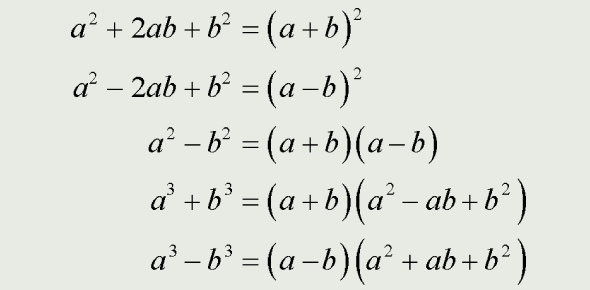# Quiz On Factoring Polynomials

10 Questions | Attempts: 6167
ShareSettingsSo, how strong is your math? Do you think your math skills are higher than any other person out there? Play a quiz on factoring polynomials that we have made for you. A polynomial is simply defined as an expression composed of variables, constants as well as exponents that are combined with the help of mathematical operations such as addition, subtraction, multiplication, and division. Wish you all the best with this quiz. Now, Let's go!

• 1.
X2 + x – 90
• A.

(x – 9)(x – 10)

• B.

(x – 9)(x + 10)

• C.

(x + 9)(x – 10)

• D.

None of these

• 2.
X+ x – 78
• A.

(x – 9)(x + 10)

• B.

(x + 4)(x + 2)

• C.

5(x - 2)(x + 2)

• D.

None of these

• 3.
X2 – 6x + 8
• A.

(x – 4)(x + 2)

• B.

(x + 4)(x + 2)

• C.

(x – 4)(x – 2)

• D.

None of these

• 4.
5x2 + 10x + 20
• A.

5(x - 2)(x + 2)

• B.

5(x + 2)(x + 2)

• C.

5(x^2 + 2x + 4)

• D.

None of these

• 5.
X2 + x – 90
• A.

(x – 9)(x – 10)

• B.

(x – 9)(x + 10)

• C.

(x + 9)(x – 10)

• D.

None of these

• 6.
X2 – 6x + 8
• A.

(x – 4)(x + 2)

• B.

(x + 4)(x + 2)

• C.

(x – 4)(x – 2)

• D.

None of these

• 7.
X+ x – 870
• A.

(x – 4)(x – 2)

• B.

(x + 4)(x + 2)

• C.

Both A & B

• D.

None of these

• 8.
5x2 + 10x + 20
• A.

5(x - 2)(x + 2)

• B.

5(x + 2)(x + 2)

• C.

5(x^2 + 2x + 4)

• D.

None of these

• 9.
2x2 + 2x – 4
• A.

2(x – 1)(x + 2)

• B.

2(x + 1)(x + 2)

• C.

(2x – 1)(x + 4)

• D.

None of these

• 10.
15x2 – 27x – 6
• A.

3(n + 1)(5n – 2)

• B.

3(5n + 1)(n – 2)

• C.

3(5n + 3)(n – 2)

• D.

None of these

## Related TopicsBack to top
×

Wait!
Here's an interesting quiz for you.